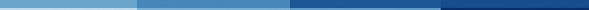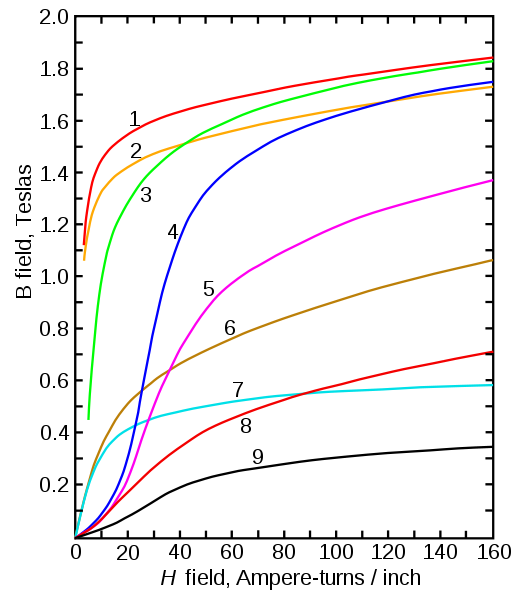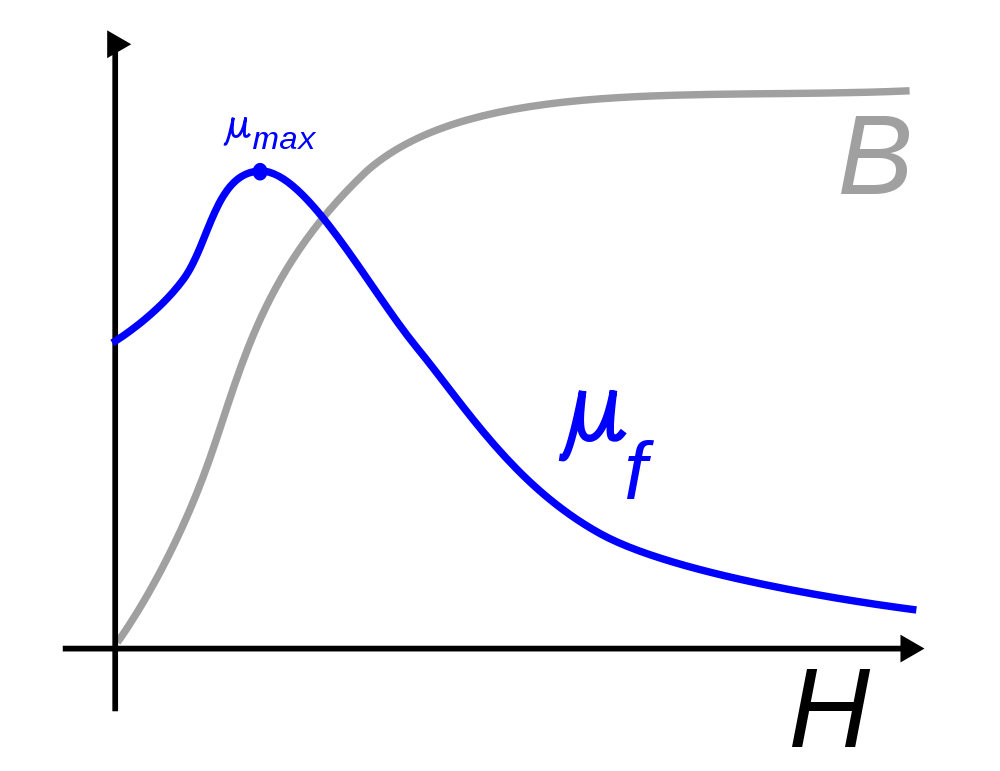AtlasCommon SOFT magnetic materialsPermeabilityFerromagnetic materialsMagnetic materialsMagnetic saturationStrongest Permanent MagnetsMagnetizing Permanent MagnetsFerrite memory reading and writingZPE EnergyMagnetic SaturationMagnetic Saturation Magnetization curves of 9 ferromagnetic materials showing saturation.Magnetic Saturation Seen in some magnetic materials, saturation is the state reached when an increase in applied external magnetizing field H cannot increase the magnetization of the material further, so the total magnetic field B levels off. It is a characteristic particularly of ferromagnetic materials, such as iron, nickel, cobalt and their alloys.-------------------Figure 1. Didaskalia-------------------Magnetization curves of 9 ferromagnetic materials, showing saturation. 1.Sheet steel, 2.Silicon steel, 3.Cast steel, 4.Tungsten steel, 5.Magnet steel, 6.Cast iron, 7.Nickel, 8.Cobalt, 9.Magnetite-------------------Figure 2. Didaskalia-------------------Due to saturation, the magnetic permeability μf of a ferromagnetic substance reaches a maximum and then declines.--------------Introduction--------------Saturation is most clearly seen in the magnetization curve (also called BH curve or hysteresis curve) of a substance, as a bending to the right of the curve (see graph at right). As the H field increases, the B field approaches a maximum value asymptotically, the saturation level for the substance. Technically, above saturation, the B field continues increasing, but at the paramagnetic rate, which is 3 orders of magnitude smaller than the ferromagnetic rate seen below saturation.The relation between the magnetizing field H and the magnetic field B can also be expressed as the magnetic permeability: μ = B / H or the relative permeability μr = μ / μ0, where μ0 is the vacuum permeability. The permeability of ferromagnetic materials is not constant, but depends on H. In saturable materials the relative permeability increases with H to a maximum, then as it approaches saturation inverts and decreases toward one.Different materials have different saturation levels. For example, high permeability iron alloys used in transformers reach magnetic saturation at 1.6 - 2.2 teslas (T), whereas ferrites saturate at 0.2 - 0.5 T. Some amorphous alloys saturate at 1.2-1.3 T.-----------------Effects and uses-----------------Saturation limits the maximum magnetic fields achievable in ferromagnetic-core electromagnets and transformers to around 2 T, which puts a limit on the minimum size of their cores. This is one reason why high power utility transformers are so large.In electronic circuits, transformers and inductors with ferromagnetic cores operate nonlinearly when the current through them is large enough to drive their core materials into saturation. This means that their inductance and other properties vary with changes in drive current. In linear circuits this is usually considered an unwanted departure from ideal behavior. When AC signals are applied, this nonlinearity can cause the generation of harmonics and intermodulation distortion. To prevent this, the level of signals applied to iron core inductors must be limited so they don't saturate. To lower its effects, an air gap is created in some kinds of transformer cores.On the other hand, saturation is exploited in some electronic devices. Saturation is employed to limit current in saturable-core transformers, used in arc welding. When the primary current exceeds a certain value, the core is pushed into its saturation region, limiting further increases in secondary current. In a more sophisticated application, saturable core inductors and magnetic amplifiers use a DC current through a separate winding to control an inductor's impedance. Varying the current in the control winding moves the operating point up and down in the saturation curve, controlling the AC current through the inductor. These are used in variable fluorescent light ballasts, and power control systems.---------------------Magnetic Saturation---------------------Is there a limit to magnetic field strength? What happens to the projectile when the coil is boosted to an extremely strong magnetic field?Are you familiar with B-H curves? First, a quick refresher about the definitions of B and H. * The magnetic field, H, tends to magnetize space. * The magnetic flux density, B, is the total magnetic effect that results.The two concepts are approximately linked with a simple equation. The magnetic flux density, B, is the total magnetic effect that results in iron: B = μ0 * μr * H, where u0 is the permeability of free space, and ur is the relative permeability of the material.The constant ur is essentially unity (= 1.0) for all materials except ferromagnetic materials, notably iron, for which the value of the relative permeability lies typically in the range 1000 to 10,000. Thus for iron, a small magnetic cause, H, creates a large magnetic effect, B.By the way, an iron/nickel compound typically has twice the constant relative permeability ( μr) as mere iron. The highest ur is "Supermalloy" at about 100,000 (see table of magnetic materials) So you might think it would perform much better than a plain iron projectile. Unfortunately, it ain't necessarily so. The total flux in a magnetic circuit is limited by any air gaps, and we've got a big one: right down the middle of the coil.B-H graph to show saturation But a more complete picture takes into account saturation. As the applied field increases, more and more magnetic domains within the ferrous material will align themselves with the external field. This temporarily makes the core act as a magnet itself, which for us will improve the forces upon the projectile. At small to medium applied fields, the flux density B is nearly linear with respect to the applied field H. But at very strong applied fields, almost all the magnetic domains are aligned.The B-H curve here illustrates the effect of magnetic saturation. It shows the effect of applying an external magnetic field to unmagnetized iron. The magnetism curve starts at the origin (0,0) and increases linearly as the magnetic field magnetizes the iron. The slope of the curve in this region is μ = μ0*μr. Eventually, however, all the magnetic domains align with the applied field, and the curve flattens out as the iron becomes magnetically saturated. As we reverse the applied magnetic field, the iron eventually becomes magnetized in the reverse direction until it again saturates.How does this relate to our projectiles? It means measurements of forces taken at small coil currents are linear with respect to applied current. As you increase current, the magnetizing force produced by an air coil is always linear, but the response in the projectile is not linear. At very large fields, the force in the projectile is not as great as linear equations would lead you to expect. The mechanical force of attraction does continue to increase, but it takes more and more coil current to achieve the same amount of increase.ConclusionSo how do you apply that general concept to a physical system? The magnetic saturation point puts a limit on the maximum effective strength to drive your coil. There will be no benefit at all if you exceed this, only wasted heat.Basically it goes like this. You need to know the exact material and dimensions of your projectile. From that, manufacturers supply the ferromagnetic permeability (or see the table of magnetic materials) and the B-H curve. From the saturation point, Bs, you know the upper limit for your design; it would be wasteful to attempt any higher flux density.You also need to compute the total reluctance R along the path of your magnetic circuit. From Bs and R you can compute the maximum flux your coil should generate. But don't worry: this upper limit is going to be huge! My initial calculation found it will be millions of ampere-turns, using any air-core coil that we've seen so far!